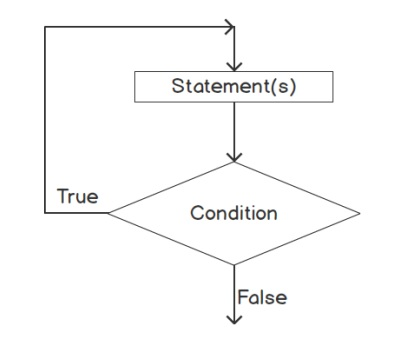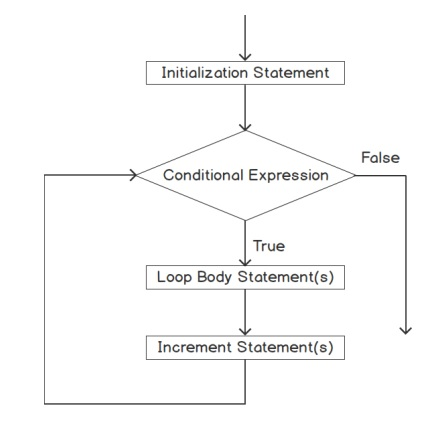# PHP Loops

Loops are control structures that allow you to execute certain statements several times, as many times as you need. Loops are used frequently in scripts to set up a block of statements that repeat.

The loop can repeat a specified number of times. The most common use is when interacting with arrays. For example, imagine you have an array with elements, but you do not know what is in it. You want to print all its elements, so you loop through all of them.

There are four types of loops:

1. While: Sets up a condition; checks the condition, and if it’s true, repeats a block of statements until the condition becomes false.

2. Do..while: Sets up a condition; executes a block of statements; checks the condition, and if it’s true, repeats the block of statements until the condition becomes false.

3. For: Sets up a counter; repeats a block of statements until the counter reaches a specified number.

4. Foreach: It is used to loop over all elements of an array.

### 1. While

The while loop is the simplest of the loops. It executes a block of code until the expression to evaluate returns false. A while loop continues repeating as long as certain conditions are true.The loop works as follows:

1. You set up a condition.
2. The condition is tested at the top of each loop.
3. If the condition is true, the loop repeats. If the condition is not true, the loop stops.

The following is the general format of a while loop:

``while (condition){  block of statements}``

Example

`\$i = 1;while (\$i < 4) {echo \$i . " ";\$i++;}`

In the example, we define a variable with value 1. Then we have a while clause in which the expression to evaluate is \$i < 4. This loop executes the content of the block of code until that expression is false. Inside the loop we are increasing the value of \$i by 1 each time, so the loop ends after 3 iterations. The output of that script will be "1 2 3". The last value printed is 3, so at that time the value of \$i was 3. After that, we increased its value to 4, so when the while clause evaluates if \$i < 4, the result is false.

Infinite Loops

It is possible to write a while loop that is infinite, that is, a loop that loops forever. If the condition never becomes false, the loop never ends. These are called infinite loops.

One of the most common problems with the while loops is creating an infinite loop. If you do not add any code inside the while loop that updates any of the variables considered in the while expression such that it can be false at some point, PHP will never exit the loop.

### 2. Do...while

A do...while loop is very similar to a while loop. Like a while loop, a do...while loop continues repeating as long as certain conditions are true. Unlike while loops, those conditions are tested at the bottom of each loop.If the condition is true, the loop repeats. When the condition is not true, the loop stops. The general format for a do..while loop is as follows:

``do{  block of statements} while (condition);``

In a while loop, the condition is checked at the top of the loop. Therefore, the loop will never execute if the condition is never true. In the do...while loop, the condition is checked at the bottom of the loop. Therefore, the loop always executes at least once, even if the condition is never true.

### 3. For

The for loop is the most complex of the four loops. It defines an initialization expression, an exit condition, and the end of an iteration expression. You set the beginning value for the counter, set the ending value, and set how the counter is incremented each time the statement block is executed.When PHP first encounters the loop, it executes what is defined as the initialization expression. Then, it evaluates the exit condition and if it resolves to true, it enters the loop. After executing everything inside the loop, it executes the end of the iteration expression. Once done, it evaluates the end condition again, going through the loop code and the end of the iteration expression, until it evaluates to false.

The general format of a basic for loop is as follows:

``for (startingvalue ; endingcondition ; increment){  block of statements;}``
• startingvalue: The starting value is a statement that sets up a variable to be your counter and sets it to your starting value. For example, the statement \$i=1; sets \$i as the counter variable and sets it equal to 1.

• endingcondition: The ending condition is a statement that sets your ending value. As long as this statement is true, the block of statements keeps repeating. When this statement is not true, the loop ends. For example, the statement \$i<10; sets the ending value for the loop to 10. When \$i is equal to 10, the statement is no longer true, and the loop stops repeating.

• increment: This statement increments your counter. For example, the statement \$i++; adds 1 to your counter at the end of each block of statements.

Example

`for (\$i = 1; \$i < 10; \$i++) { echo \$i . " ";}`

The initialization expression is \$i = 1, and is executed only the first time. The exit condition is \$i < 10, and it is evaluated at the beginning of each iteration. The end of the iteration expression is \$i++, which is executed at the end of each iteration. This example prints the numbers from 1 to 9.

### 4. Foreach

The foreach loop is used to loop over all elements of an array. This loop is exclusive for arrays, and it allows you to iterate an array entirely, even if you do not know its keys. There are two options for the syntax.

`foreach (\$array as \$value) {   block of statements  echo \$value . " ";}`

or

`foreach (\$array as \$key => \$value) {   block of statements  echo \$key . " -> " . \$value . " ";}`

The number of times loop is executed is equal to the number of elements in the \$array array. The foreach loop accepts an array and it specifies a variable which will contain the value of the entry of the array. Optionally, you can specify a variable that contains the key of each iteration, as in the second loop.

The foreach loops are also useful with maps, where the keys are not necessarily numeric. The order in which PHP iterates the array will be the same order that you used to insert the contents in the array.

### Breaking out of Loop

Sometimes you want your script to break out of a loop. PHP provides two statements for this purpose:

break: Breaks completely out of a loop and continue with the script statements after the loop.

continue: Skips to the end of the loop where the condition is tested. If the condition tests positive, the script continues from the beginning of the next iteration.